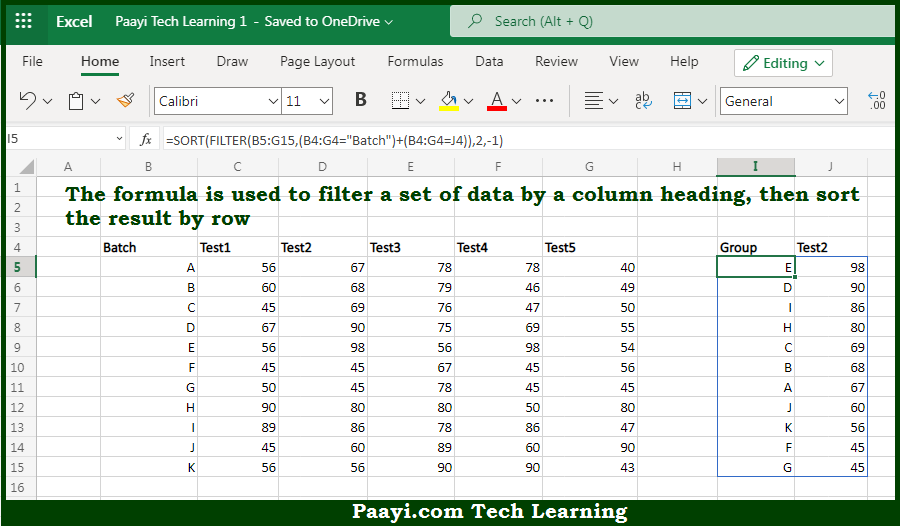# Learn How to Filter By Column And Sort By Row in Microsoft Excel

Written by | 0 Comments | 525 Views

In this article, you will learn how to evaluate things in Dynamic Arrays with formulas in Microsoft Excel using a single/combination(s) of functions. You will also know How toFilter By Column And Sort By Row and see the generic formula.

How to Filter By Column And Sort By Row in Microsoft Excel

The main purpose of this formula is to filter a set of data by a column heading, then sort the result by row. Here we will learn how to filter by column and sort by row from a given data range in the workbook in Microsoft Excel. That implies, with the help of a formula based on the FILTER and SORT function you can able to filter a set of data by a column heading, then sort the result by row. So, with the help of this formula, you can able to filter by column and sort by row from a given data range in the workbook in Microsoft Excel.

General Formula to Filter By Column And Sort By Row

The Explanation for the Filter By Column And Sort By RowSo we know that with the help of the given formula above you can able to filter a set of data by a column heading, then sort the result by row. Here we will learn how to filter by column and sort by row from a given data range in the workbook in Microsoft Excel. As we know that the FILTER function is used to filter data vertically, selecting rows that match provided conditions. It should be noted that the FILTER function can also select data horizontally. The crucial thing is to provide logic for the included argument that will return a horizontal array with the same number of columns as the source data. So, with the help of this formula, you can able to filter a set of data by a column heading, then sort the result by row. Here we will learn how to filter by column and sort by row from a given data range in the workbook in Microsoft Excel.